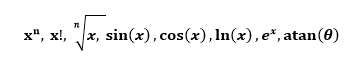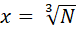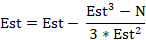# We Helped With This Engineering Homework: Have A Similar One?

SOLVEDCategory Engineering Other Undergraduate Solved Engineering Homework Solutions

## Assignment Description

ENED 1090 HW #7

Due, Friday, November 10, 2017 at 5:00pm

Problem 1:   Consider the code show below.  Complete the table showing the values for the variables as the loop progresses.  Do this without MATLAB first then check your results using MATLAB.

x = 2;

y = 1;

for k = 1:5

z = x - y;

x = y;

y = z;

end

 z x y Before Loop Not Defined Before Loop 2 1 k = 1 k = 2 k = 3 k = 4 k = 5

Problem 2Consider the code show below.  Complete the table showing the values for the variables as the loop progresses. Do this without MATLAB first then check your results using MATLAB.

t = [ 3 -7 -2  7  3 -8 ];

c = 0;

for k = 1:length(t)

if abs(t(k)) < 5

c = c + 1;

location(c) = k;

end

end

 c location Before Loop 0 Not Defined Before Loop k = 1 k = 2 k = 3 k = 4 k = 5 k = 6

Problem 3: Numerical Algorithm for Determining Roots

The only mathematical operations the processor in a computing device (calculator, computer, cell phone, etc.) can perform are addition, subtraction, multiplication, and division.  With this rather limited set of operations, how does your calculator (or Excel and MATLAB) determine values for more complicated mathematical operations such as:To calculate values for these functions, an iterative algorithm or a numerical method that only requires the basic arithmetic operations of addition, subtraction, multiplication, and division is needed.  In this problem, you will look at a very simple algorithm for computing the cube root of a number based on the Newton-Raphson algorithm.

The algorithm is very simple.  We want to find• Make an initial guess for x:    Est
• See how close you are:           Error = abs(Est^3 – N)
• If the Error is too big, update the estimate as follows:• Recalculate the Error using the new estimate
• Repeat this process to obtain a better guess

Write a MATLAB script that does the following:

1. Prompts the user for the Number, N, for which he or she wishes to find the cube root
2. Prompt the user for the number of iterations he or she wishes to perform
3. Set the initial guess to 1
4. Uses the algorithm describe above to repeatedly update the estimate and error for the number of times specified by the user
5. Saves all the values for Est in a vector
6. Outputs the following:

·         The final value of the estimate for the cube root of N to 4 decimal places

·         The final Error to 4 decimal places

·         A plot with all the values for Est on the y axis and the iteration value on the x axis

Use your script to complete the table below.  Paste your plot for the last row of the table where indicated.

 N Iterations Final Estimate Final Error 3375 10 3375 12 3375 14 3375 16

PASTE PLOT HERE:

PASTE MATLAB CODE HERE:

Problem 4: Data Processing

As you’ve seen in labs 8 and 9, one of the benefits of using for loops is to easily process large amounts of data.  For this problem, you will be looking at data for the value (in US \$) of goods imported and exported for countries around the world in 2016.  This information is found in the HW7_P4.xlsx file.  Write a script in MATLAB that will do the following:

·         Loads in the import data and export data from the Excel file using the xlsread command (you should end up with 2 vectors, one for import data and one for export data):
Example: data = xlsread('filename','range of cells');

·         Process all of the data to determine the following values:

o   Number of countries with more exports than imports

o   Number of countries with more imports than exports

o   Number of countries that have the same number of imports and exports

o   The maximum and minimum value of imports among the countries

o   The maximum and minimum value of exports among the countries

·         Display all requested values with appropriate units

PASTE SCRIPT OUTPUT HERE:

PASTE MATLAB CODE HERE:

Once you have completed the assignment, upload this Word document and any MATLAB m-files to Blackboard.

Is it free to get my assignment evaluated?

Yes. No hidden fees. You pay for the solution only, and all the explanations about how to run it are included in the price. It takes up to 24 hours to get a quote from an expert. In some cases, we can help you faster if an expert is available, but you should always order in advance to avoid the risks. You can place a new order here.

How much does it cost?

The cost depends on many factors: how far away the deadline is, how hard/big the task is, if it is code only or a report, etc. We try to give rough estimates here, but it is just for orientation (in USD):

 Regular homework \$20 - \$150 Advanced homework \$100 - \$300 Group project or a report \$200 - \$500 Mid-term or final project \$200 - \$800 Live exam help \$100 - \$300 Full thesis \$1000 - \$3000

How do I pay?

Credit card or PayPal. You don't need to create/have a Payal account in order to pay by a credit card. Paypal offers you "buyer's protection" in case of any issues.

Why do I need to pay in advance?

We have no way to request money after we send you the solution. PayPal works as a middleman, which protects you in case of any disputes, so you should feel safe paying using PayPal.

Do you do essays?

No, unless it is a data analysis essay or report. This is because essays are very personal and it is easy to see when they are written by another person. This is not the case with math and programming.

Why there are no discounts?

It is because we don't want to lie - in such services no discount can be set in advance because we set the price knowing that there is a discount. For example, if we wanted to ask for \$100, we could tell that the price is \$200 and because you are special, we can do a 50% discount. It is the way all scam websites operate. We set honest prices instead, so there is no need for fake discounts.

Do you do live tutoring?

No, it is simply not how we operate. How often do you meet a great programmer who is also a great speaker? Rarely. It is why we encourage our experts to write down explanations instead of having a live call. It is often enough to get you started - analyzing and running the solutions is a big part of learning.

What happens if I am not satisfied with the solution?

Another expert will review the task, and if your claim is reasonable - we refund the payment and often block the freelancer from our platform. Because we are so harsh with our experts - the ones working with us are very trustworthy to deliver high-quality assignment solutions on time.

## Popular Solved Assignments Like This

Customer Feedback

"Thanks for explanations after the assignment was already completed... Emily is such a nice tutor! "

Order #13073